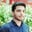Related Tags

functions
objective c
communitycreator

# What are functions in Objective C?Salman Yousaf

### Overview

A functionAlso called procedure, subroutine and method. contains a group of statements that work together to perform a specific task. In Objective-C, all the programs contain a C function that is main(). We can also divide the code into different functions. Each performs a specific task.

In a function declaration, the name of the function, its return type, and parameters are specified. Function definition contains the body of the function. There is also a variety of built-in functions available in Objective-C. For example, the append string method is used to append a string to another string.

### Syntax

 -(return type) method_name: (argument_Type1) argument_Name1
joining_argument2: (argument_Type2) argument_Name2 …… {
Function body
}
Syntax of a function

### Parts of a function

• Return type: The return type is the data type of the value that is returned by the method. Some functions perform a specific task without returning any value. The return type of that method is defined as void.
• Method name: It shows the name of the function. The name of the function and a list of its parameter together create the method signature.
• Arguments: The argument acts just like a placeholder. We pass a value to the function’s argument when the method is called.
• Joining parameter: Joining parameter assists in reading it clearly and easily when we call it.
• Method body: It consists of the number of statements that define the tasks to be performed by the function.

### Example

#import <Foundation/Foundation.h>
@interface TestClass:NSObject

/*function declaration */
- (int)sum:(int)n1 andN2:(int)num2;
@end
@implementation TestClass

/* function returning the sum of two numbers */
- (int)sum:(int)n1 andN2:(int)n2 {

/* local variable declaration */
int ans;
ans=n1+n2;
return ans;
}
@end
int main () {

/* local variable definition */
int x = 500;
int y = 150;
int r;
TestClass *testClass = [[TestClass alloc]init];

/* calling a method to get the sum of two numbers */
r = [testClass sum:x andN2:y];

NSLog(@"The sum is : %d\n", r );
return 0;
}

### Explanation

• Line 5: We declare the function for calculating the sum of two numbers.
• Line 10: We define the function body here. The two numbers are added and the result is returned.
• Line 21–23: We define local variables that are passed as parameters to the function.
• Line 27: We call the method to compute the sum of two numbers.

RELATED TAGS

functions
objective c
communitycreator

CONTRIBUTORSalman Yousaf
RELATED COURSES

View all Courses

Keep Exploring

Learn in-demand tech skills in half the time